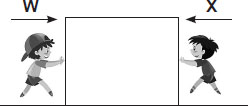# User Forum

Subject :NSO    Class : Class 3

Refer to the given figure and select the correct option.A If W = X, box will move towards W.
B If W > X, the box will move towards X.
C If W = X, the box will move towards X.
D If W < X, the box will move towards X.

Class : Class 3

Class : Class 3

Class : Class 4

## Ans 4:

Class : Class 5
The correct answer is B because ; When a force is applied , the object moves farther from the source of force . In case of opposing forces the object moves towards the weaker force . In case both the forces are equal, the object shows no movement at all.

Class : Class 3
B

Class : Class 4